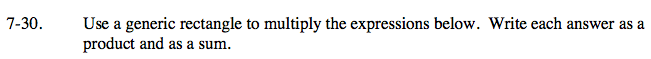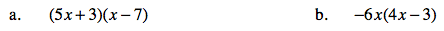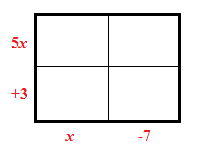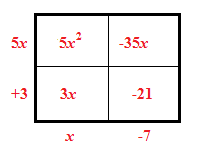### Home > AC > Chapter 7 > Lesson 7.1.3 > Problem7-30

7-30.
1. Use a generic rectangle to multiply the expressions below. Write each answer as a product and as a sum. Homework Help ✎

1. (5x + 3)(x − 7)

2. −6x(4x − 3)Draw a generic rectangle with the dimensions given.

Use the method from part (a).

Multiply to find the area of each small rectangle.

Add the terms inside the rectangle to write the product as a sum.(5x + 3)(x − 7) = 5x2 + 3x − 35x − 21